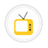you are here->home->Physics->Class 10
Class 10
Class 10 Physics experimentsTo study refraction of light in rectangular glass slabTo trace the path of a ray of light passing through a rectangular glass slab for different angles of incidence. Measure the angle of incidence, angle of refraction, angle of emergence and interpret the result.To study reflection in concave mirrorTo study reflection in concave mirror and observe image formation in different positions of the objectTo find focal length of a Concave MirrorTo determine the focal length of Concave mirror by obtaining the image of a distant objectVerification of Hooke's LawHooke's law of elasticity is an approximation that states that the extension of a spring is in direct proportion with the load applied to it.To study the field lines formed around a bar magnetThis experiment is to study the magnetic field produced by a bar magnet. This is done by observing the arrangement of Iron Filing and behavior of magnetic compass around the Magnet .Verification of Ohm's LawTo study the dependence of potential difference (V) across a resistor on the current (I) passing through it and to determine its resistance. Also plot a graph between V and IMagnetic field lines around current carrying conductorThis is an activity to observe the magnetic effects of current.The magnetic field lines around current carrying solenoid.This experiment is a special case of the fundamental fact that a current carrying conductor has a magnetic field around it. Here we are modelling the behavior and properties of the magnetic field when the conductor takes the shape of a loopForce on a current carrying conductor in a magnetic fieldTo verify that this behavior is accurately described by Fleming’s Left Hand Rule.Electromagnetic InductionTo observe how a moving magnet can be used to generate electric current. To verify that the behavior is accurately described by Faraday's law.